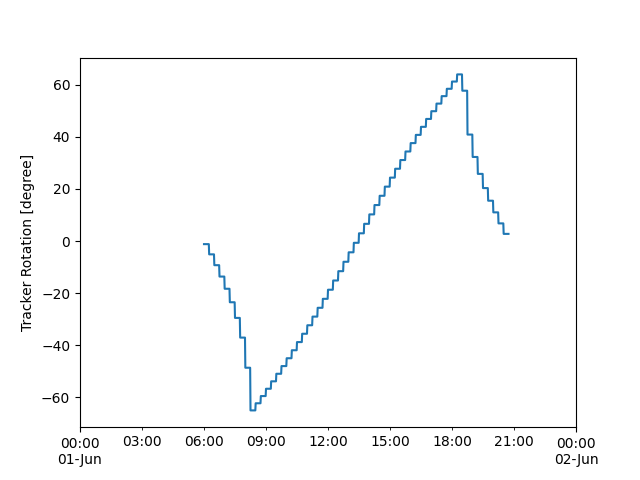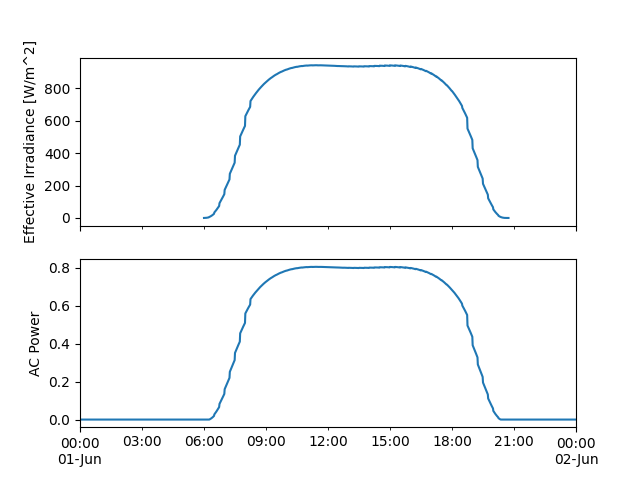# Discontinuous Tracking¶

Example of a custom Mount class.

Many real-world tracking arrays adjust their position in discrete steps rather than through continuous movement. This example shows how to model this discontinuous tracking by implementing a custom Mount class.

from pvlib import tracking, pvsystem, location, modelchain
from pvlib.temperature import TEMPERATURE_MODEL_PARAMETERS
import matplotlib.pyplot as plt
import pandas as pd


We’ll define our custom Mount by extending SingleAxisTrackerMount for convenience. Another approach would be to extend AbstractMount directly; see the source code of SingleAxisTrackerMount and FixedMount for how that is done.

class DiscontinuousTrackerMount(pvsystem.SingleAxisTrackerMount):
# inherit from SingleAxisTrackerMount so that we get the
# constructor and tracking attributes (axis_tilt etc) automatically

def get_orientation(self, solar_zenith, solar_azimuth):
# Different trackers update at different rates; in this example we'll
# assume a relatively slow update interval of 15 minutes to make the
# effect more visually apparent.
zenith_subset = solar_zenith.resample('15min').first()
azimuth_subset = solar_azimuth.resample('15min').first()

tracking_data_15min = tracking.singleaxis(
zenith_subset, azimuth_subset,
self.axis_tilt, self.axis_azimuth,
self.max_angle, self.backtrack,
self.gcr, self.cross_axis_tilt
)
# propagate the 15-minute positions to 1-minute stair-stepped values:
tracking_data_1min = tracking_data_15min.reindex(solar_zenith.index,
method='ffill')
return tracking_data_1min


Let’s take a look at the tracker rotation curve it produces:

times = pd.date_range('2019-06-01', '2019-06-02', freq='1min', tz='US/Eastern')
loc = location.Location(40, -80)
solpos = loc.get_solarposition(times)
mount = DiscontinuousTrackerMount(axis_azimuth=180, gcr=0.4)
tracker_data = mount.get_orientation(solpos.apparent_zenith, solpos.azimuth)
tracker_data['tracker_theta'].plot()
plt.ylabel('Tracker Rotation [degree]')
plt.show()With our custom tracking logic defined, we can create the corresponding Array and PVSystem, and then run a ModelChain as usual:

module_parameters = {'pdc0': 1, 'gamma_pdc': -0.004, 'b': 0.05}
temp_params = TEMPERATURE_MODEL_PARAMETERS['sapm']['open_rack_glass_polymer']
array = pvsystem.Array(mount=mount, module_parameters=module_parameters,
temperature_model_parameters=temp_params)
system = pvsystem.PVSystem(arrays=[array], inverter_parameters={'pdc0': 1})
mc = modelchain.ModelChain(system, loc, spectral_model='no_loss')

# simple simulated weather, just to show the effect of discrete tracking
weather = loc.get_clearsky(times)
weather['temp_air'] = 25
weather['wind_speed'] = 1
mc.run_model(weather)

fig, axes = plt.subplots(2, 1, sharex=True)